This handy Math in Focus Grade 4 Workbook Answer Key Chapter 13 Symmetry detailed solutions for the textbook questions.

Math Journal

Complete.

Question 1.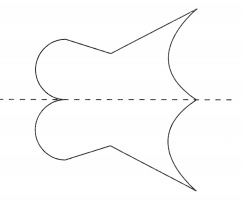Explain why the dotted line is the line of symmetry for the figure.
If a figure can be folded or divided into half so that the two halves match exactly then such a figure is called a symmetric figure. The figure above is symmetric.
The dotted line in above symmetric figure that divides the figure into two equal halves is called the line of symmetry.

Question 2.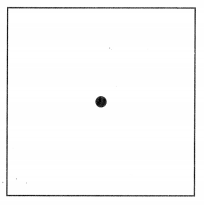Does the square have rotational symmetry about the center shown? Explain.
Yes, The square have rotational symmetry for the center shown in the above figure.
Explanation:
If a figure is rotated around a center point and it still appears exactly as it did before the rotation, it is said to have rotational symmetry. A number of shapes like squares, circles, regular hexagons etc. have rotational symmetry.

Challenging Practice

Shade the correct squares so that the pattern of shaded squares has line symmetry about the given dotted line.

Question 1.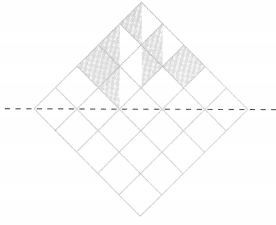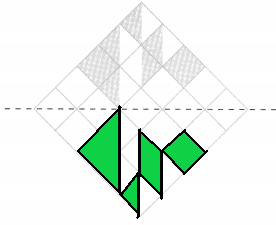Shaded the correct squares in the above figure. The pattern of shaded squares has line symmetry for the given dotted line.

Question 2.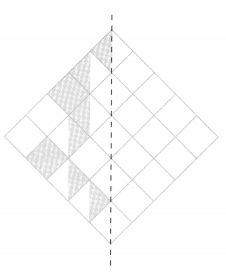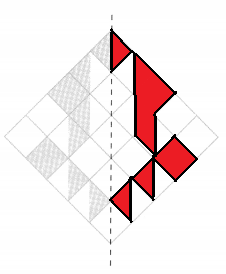Shaded the correct squares in the above figure. The pattern of shaded squares has line symmetry for the given dotted line.

In the square grid below, design a symmetric pattern that has both line symmetry about the given dotted line and rotational symmetry.

Question 3.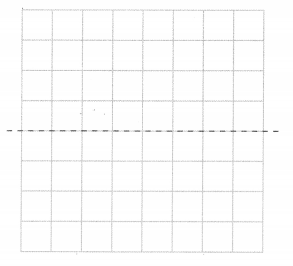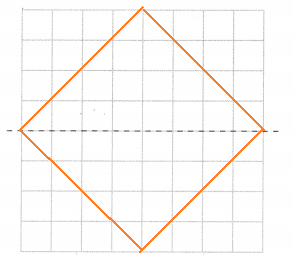In the above square grid, designed a symmetric pattern that has both line symmetry for the given dotted line and rotational symmetry.

Problem Solving

Add one unit square to each figure to make it symmetric about the given dotted line.

Question 1.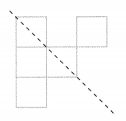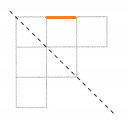Added one unit square to the above figure to make it symmetric for the given dotted line.

Question 2.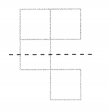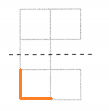Added one unit square to the above figure to make it symmetric for the given dotted line.

Shade unit squares in each pattern so that it has line symmetry and rotational symmetry.

Question 3.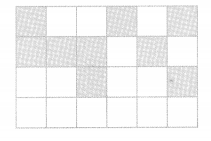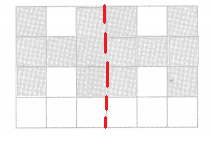Shaded unit squares in above pattern. Now the above figure has both line symmetry and rotational symmetry.

Question 4.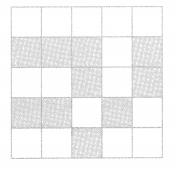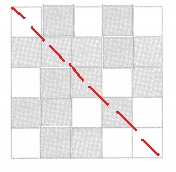Shaded unit squares in above pattern. Now the above figure has both line symmetry and rotational symmetry.

Solve.

Question 5.
Using the digits 0, 1, 6, 8, and 9, write down all the possible three-digit numbers that have rotational symmetry. The digits can be used more than once.